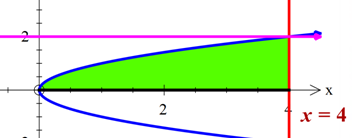# Evaluate the integral by reversing the order of integration. \int_{0}^{2}\int_{y^2}^{4} ycos(x^2)...

## Question:

Evaluate the integral by reversing the order of integration.

{eq}\int_{0}^{2}\int_{y^2}^{4} ycos(x^2) {/eq} dxdy

## Double Integral:

Let us first draw the graph of the region. Then we will find the limits of integration where the limits of y will be variable and the limits of x are constant.To solve the integral we will proceed as

{eq}\int_{0}^{2}\int_{y^{2}}^{4}y\cos x^{2}dydx {/eq}

By reversing the order of integration we will proceed as

{eq}\int_{0}^{4}\int_{0}^{\sqrt{x}}y\cos x^{2}dydx {/eq}

Integrating with respect to y we get

{eq}\int_{0}^{4}\frac{x}{2}\cos x^{2}dx {/eq}

Integrating with respect to x

{eq}\frac{1}{4}\left [\sin x^{2} \right ]_{0}^{16}\\ =\frac{1}{4}\sin 16 {/eq}